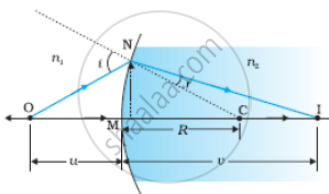# A Point-object is Placed on the Principal Axis of Convex Spherical Surface of Radius of Curvature R, Which Separates the Two Media of Refractive Indices N1 and N2 (N2>N1). - Physics

Diagram

A point-object is placed on the principal axis of convex spherical surface of radius of curvature R, which separates the two media of refractive indices n1 and n2 (n2>n1). Draw the ray diagram and deduce the relation between the distance of the object (u), distance of the image (v) and the radius of curvature (R for refraction to take place at the convex spherical surface from rarer to denser medium.

#### Solution

(a)Refraction at a spherical surface separating two media.

The figure shows the geometry of the formation of the image I of an object O on the principal axis of a spherical surface with center of curvature C, and radius of curvature R. The rays are incident from a medium of refractive index n1, to another of refractive index n2. As before, we take the aperture (or the lateral size) of the surface to be small compared to other distances involved, so that small angle approximation can be made. In particular, NM will be taken to be nearly equal to the length of the perpendicular from the point N on the principal axis.

We have, for small angles,

tan ∠NOM = "MN"/"OM"

tan ∠NCM = "MN"/"MC"

tan ∠NIM = "MN"/"MI"

Now, for ΔNOC, is the exterior angle. Therefore i = ∠NOM + ∠NCM

i = "MN"/"OM" + "MN"/"MC"................(1)

Similarly,
r = ∠NCM - ∠NIM

i.e., r = "MN"/"MC" - "MN"/"MI"..............(2)

Now, by Snell’s law
n1sin i = n2sin r
or for small angles
n1i = n2r
Substituting i and r from Eqs.(1) and (2), we get

"n"_1/"OM" + "n"_2/"MI" = ("n"_2-"n"_1)/"MC"............(3)

Here, OM, MI, and MC represent magnitudes of distances. Applying the Cartesian sign convention,

OM = – u, MI = + v, MC = + R

Substituting these in Eq.(3), we get
"n"_2/"v" - "n"_1/"u" = ("n"_2-"n"_1)/"R"..................(4)

Equation (4) gives us a relation between object and image distance in terms of the refractive index of the medium and the radius of curvature of the curved spherical surface. It holds for any curved spherical surface.

Concept: Rarer and Denser Medium
Is there an error in this question or solution?